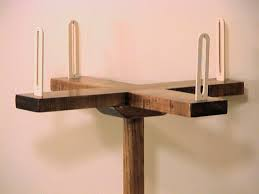This type of survey is undertaken to locate boundaries of a field or a field or plat and determinations of its area.

Principle:

The principle of the survey is to divide the given area in to no of right angled triangles and trapezoids and to calculate and plot the areas of triangles and trapezoids.

Instruments/Material Required:

Two Chains, arrows taps, ranging rods, cross-staff and drawing material

Procedure:

Two chains are usually provided one for measuring distance along the chain line and other for measuring the offsets. The cross staff is used to set out the perpendicular directions for offsets. In this survey, the base line runs through the center of the area, so that the offsets are left or right side of base line are fairly equal. To check accuracy length of the boundary lines may also be measured. After the field work is over, the survey is plotted to a suitable scale.

Calculations of Field Area:

By this method of survey, the field as divided in to right angled triangles and trapezoids are calculated as under:

1. Area of right -angled triangle = ½ base x Height.

2. Area of trapezoid= sum of parallel sides/2 x Height.

Add the areas of all the triangles & trapezoids and sum is equal to the total of a field.

The computations for area should be written in a tabular from as given below.

TABLE

 Serial no. Figure Chain age in m base in m Offset in m Mean offset in m Areas in m2 A=col. 4.xcol.6 1 2 3 4 5 6 7 Total m2

Source